Next: Second Order Sufficient Conditions Up: Sufficient Optimality for Discretized Previous: Parabolic and Elliptic Control

# Known SSC Results for the Continuous Problems

No attempt will be made to quote all relevant results on optimality conditions. In the case of problem (P) without state constraints the following set of SSC is stated in . First, the Lagrange function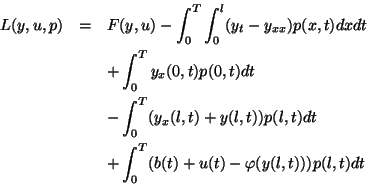is defined with the Lagrange multiplier function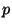. Then the second derivative of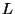with respect to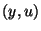is called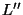and is evaluated at a point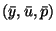satisfying the first order optimality conditions. The key requirement is the inequality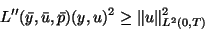(3.1)

which has to hold for allwhich satisfy the linearized (at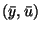) constraints. In the example considered in  and below, the inequality even holds for all. Locally, then,is a minimizer of problem (P).

SSC have not been stated exactly for the elliptic control problems (EB) in the previous section, but a series of papers address special cases. In , for example, a good overview of the literature is given and the boundary control problem covered in much technical detail is nearly identical to (EB) except for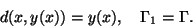Finally, the state-constrained case is addressed in  for (EB) and in  for (P).Next: Second Order Sufficient Conditions Up: Sufficient Optimality for Discretized Previous: Parabolic and Elliptic Control
Hans Mittelmann
2000-08-31